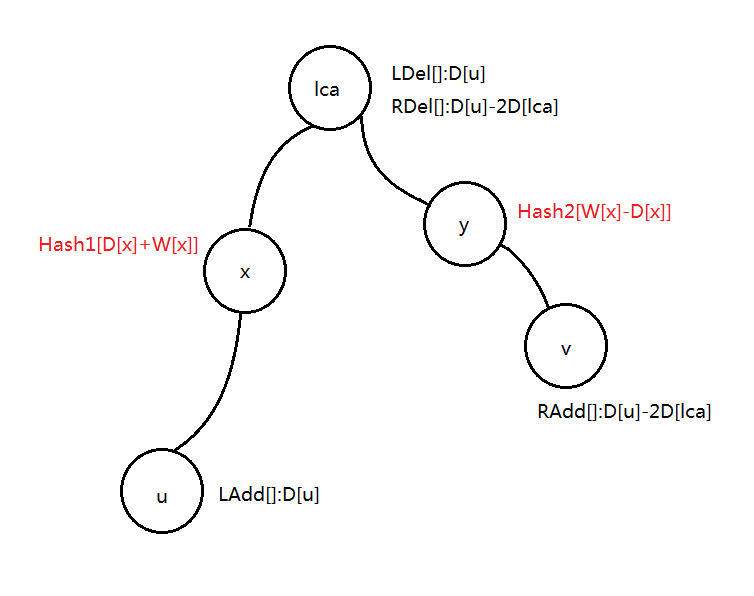「NOIP2016」天天爱跑步 - 树上Hash表 | Bill Yang's Blog

初步想法

①对于左链（即从开始结点$u$走到$lca$）

②让我们再看看右链（即从$lca$走到结束结点$v$）

数据结构

• $LAdd[i][]$：$i$结点左链的添加数值
• $LDel[i][]$：$i$结点左链的删除数值
• $RAdd[i][]$：$i$结点右链的添加数值
• $RDel[i][]$：$i$结点右链的删除数值

• $Hash1[x]$：左链上数值为x的有多少个
• $Hash2[x]$：右链上数值为x的有多少个0%